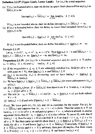# Upper and Lower Linits (lim sup and lim inf) - Sohrab Proposition 2.2.39 (b) ....

Gold Member
MHB
I am reading Houshang H. Sohrab's book: "Basic Real Analysis" (Second Edition).

I am focused on Chapter 2: Sequences and Series of Real Numbers ... ...

I need help with the proof of Proposition 2.2.39 (b)

Proposition 2.2.39 (plus definitions of upper limit and lower limit ... ) reads as follows:

View attachment 9244

Can someone please demonstrate a formal and rigorous proof of Part (b) of Proposition 2.2.39 ...

Help will be appreciated ... ...

Peter

#### Attachments

•Sohrab - Proposition 2.2.39 ... .png
50.2 KB · Views: 33

Gold Member
I presume you know monotone convergence theorem...

$u_n$ is a monotone non-decreasing sequence and the sequence is bounded, so it has a limit $L_u$
$v_n$ is a monotone non-increasing sequence and is bounded so it has a limit $L_v$

but for all $n$
$u_n \leq v_n$
by construction hence passing limits,
$L_u \leq L_v$
(This is another basic property of limits... if you aren't familiar, argue by contradiction that $L_u - L_v = c \gt 0$, now select something easy, say $\epsilon := \frac{c}{10}$ which implies there is some $N$ such that for all $n\geq N$ in each sequence (a slightly more careful approach is $N =\max\big(N_v, N_u\big)$)

you have
====
$\vert u_n - L_v\vert \lt \epsilon$
edit: to cleanup a typo, this should have said: $\vert u_n - L_u\vert \lt \epsilon$ . I had the wrong subscript.
====
and $\vert v_n - L_v\vert \lt \epsilon$ but this implies $u_n \gt v_n$ which is a contradiction -- sketching this out is best... it implies that $v_n \lt L_v + \frac{1}{10}c \lt L_v + \frac{9}{10}c = L_v + c - \frac{1}{10}c = L_u- \frac{1}{10}c \lt u_n$ )

Last edited:
Gold Member
MHB
I presume you know monotone convergence theorem...

$u_n$ is a monotone non-decreasing sequence and the sequence is bounded, so it has a limit $L_u$
$v_n$ is a monotone non-increasing sequence and is bounded so it has a limit $L_v$

but for all $n$
$u_n \leq v_n$
by construction hence passing limits,
$L_u \leq L_v$
(This is another basic property of limits... if you aren't familiar, argue by contradiction that $L_u - L_v = c \gt 0$, now select something easy, say $\epsilon := \frac{c}{10}$ which implies there is some $N$ such that for all $n\geq N$ in each sequence (a slightly more careful approach is $N =\max\big(N_v, N_u\big)$)

you have $\vert u_n - L_v\vert \lt \epsilon$ and $\vert v_n - L_v\vert \lt \epsilon$ but this implies $u_n \gt v_n$ which is a contradiction -- sketching this out is best... it implies that $v_n \lt L_v + \frac{1}{10}c \lt L_v + \frac{9}{10}c = L_v + c - \frac{1}{10}c = L_u- \frac{1}{10}c \lt u_n$ )

Thanks for the help, steep ...

Just reflecting on what you have written...

Peter